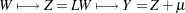Unconditional Simulation

It is a simple matter to produce an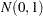random number, and by stackingsuch numbers in a column vector you obtain a vector with independent standard normal components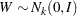. The meaning of the terms independence and randomness in the context of a deterministic algorithm required for the generation of these numbers is somewhat subtle; see Knuth (1973, Vol. 2, Chapter 3) for a discussion of these issues.

Rather than, what is required is the generation of a vector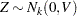—that is,with covariance matrix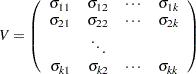where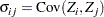If the covariance matrix is symmetric and positive definite, it has a Cholesky root
such that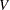can be factored as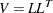whereis lower triangular. See Ralston and Rabinowitz (1978, Chapter 9, Section 3-3) for details. This vector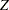can be generated by the transformation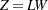. Note that this is where the assumption of multivariate normality is crucial. If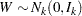, thenis also normal or Gaussian. The mean ofis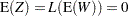and the variance is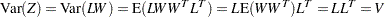Finally, let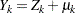; that is, you add a mean term to each variable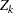. The covariance structure of the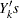remains the same. Unconditional simulation is done by simply repeatedly generatingrandom numbers, stacking them, and performing the transformation